## Table of ContentOpen Access

ARTICLE

# Short-Term Power Load Forecasting with Hybrid TPA-BiLSTM Prediction Model Based on CSSA

Jiahao Wen, Zhijian Wang*

School of Information Science, Guangdong University of Finance and Economics, Guangzhou, China

* Corresponding Author: Zhijian Wang. Email:(This article belongs to this Special Issue: Models of Computation: Specification, Implementation and Challenges)

Computer Modeling in Engineering & Sciences 2023, 136(1), 749-765. https://doi.org/10.32604/cmes.2023.023865

## Abstract

Since the existing prediction methods have encountered difficulties in processing the multiple influencing factors in short-term power load forecasting, we propose a bidirectional long short-term memory (BiLSTM) neural network model based on the temporal pattern attention (TPA) mechanism. Firstly, based on the grey relational analysis, datasets similar to forecast day are obtained. Secondly, the bidirectional LSTM layer models the data of the historical load, temperature, humidity, and date-type and extracts complex relationships between data from the hidden row vectors obtained by the BiLSTM network, so that the influencing factors (with different characteristics) can select relevant information from different time steps to reduce the prediction error of the model. Simultaneously, the complex and nonlinear dependencies between time steps and sequences are extracted by the TPA mechanism, so the attention weight vector is constructed for the hidden layer output of BiLSTM and the relevant variables at different time steps are weighted to influence the input. Finally, the chaotic sparrow search algorithm (CSSA) is used to optimize the hyperparameter selection of the model. The short-term power load forecasting on different data sets shows that the average absolute errors of short-term power load forecasting based on our method are 0.876 and 4.238, respectively, which is lower than other forecasting methods, demonstrating the accuracy and stability of our model.

## Keywords

1  Introduction

The power system presents a market-oriented trend, and the accurate forecasting of power loads is one of its key tasks . Load forecasting data is the basis of power system dispatching, and the improvement in its accuracy is vital for promoting the development of the power system .

The commonly used methods for short-term power load forecasting fall into two main categories: traditional time series forecasting models and machine learning forecasting models. The time series forecasting models, such as the exponential smoothing analysis , multiple linear regression [4,5], and regression analysis , speculate the future load changes based on the load data of the research variables and their change patterns. Although these models are simple, they are ineffective in excluding the influence of external factors, and their nonlinear fitting abilities are also unsatisfying.

Nevertheless, the emergence of machine learning prediction models, like neural networks , support vector machines (SVM) [10,11], and random forests (RF) , has effectively solved the nonlinear relationship between data. Neural networks can realize the deep mining of load time series and achieve high prediction accuracy. For example, the attention mechanism is used to assign weights to the input features of the BiLSTM neural network to build a more effective prediction model to improve prediction accuracy. Based on the attention mechanism, Wei et al.  improved the influence of important information by giving different weights to the hidden states of convolutional neural networks and long-short-term memory neural networks. The temporal pattern attention (TPA) mechanism extracts important information about different features at different time steps by performing convolution operations on hidden state features . Wang et al.  proposed a bi-directional long short-term memory (BiLSTM) neural network model based on the TPA mechanism, which weights related variables from different time steps to extract the complex internal relations between different influencing factors. The traditional attention mechanism examines information at the previous time step and selects relevant information to help generate the output, but it cannot capture time patterns across multiple time steps. Herein, we apply the temporal pattern attention (TPA) mechanism to improve the accuracy of power load fore-casting by mining the complex internal relationship between multiple time steps and different variables.

The central idea of the swarm intelligence optimization algorithm is to search for the optimal solution in the solution space within a specific range by simulating bionics . The most widely used swarm optimization algorithms are the sparrow search algorithm (SSA), black widow optimization algorithm (BWOA), whale optimization algorithm (WOA), mayfly optimization algorithm (MA), etc. The SSA, an emerging me-ta-heuristic algorithm proposed in 2020, can achieve hyperparameter optimization by constantly updating the individual location of the sparrow and simulating the foraging and anti-predation behavior of the sparrow. The chaotic sparrow search algorithm  and the improved particle swarm optimization algorithm (IPSO)  are used to optimize the model network in this paper. The basis of the sparrow search algorithm introduces Tent chaotic search and Gaussian mutation, which increases the population diversity, improves the algorithm’s search performance and development performance and avoids falling into local optimum. The chaotic sparrow search algorithm has higher solution accuracy than the sparrow search algorithm. Due to the limited global optimization ability and convergence speed of the basic PSO, the improved particle swarm algorithm uses nonlinear inertia weight to improve the performance of the particle swarm. The chaotic sparrow search algorithm optimized network model and improved particle swarm optimization algorithm optimized network model are compared and analyzed, which proves that the chaotic sparrow search algorithm optimized network model has better prediction performance.

Herein, we propose a bidirectional long short-term memory (BiLSTM-TPA) neural network prediction method based on temporal attention for short-term power load forecasting. Considering the inner relationship between multivariate and time series, the grey correlation analysis method is used to determine a similar sample set with a large correlation degree with the day to be predicted to ease neural network prediction. Then, the positive and negative internal characteristics of power load data are learned through the bidirectional LSTM layer, and the TPA temporal pattern attention mechanism is combined to further learn the interdependence between multiple variables at different times and sequences. Finally, the CSSA chaotic sparrow search algorithm is used to optimize the hyper-parameters of the BiLSTM-TPA model to obtain the final prediction results. The proposed prediction model has a decrease in the mean absolute percentage error, root mean square error, and mean absolute error by analyzing the training results of load data in different regions of China and comparing it with other prediction algorithms.

2  Methods

2.1 Gray Correlation Analysis

The grey correlation is a measure of the magnitude of the association between two or more factors, and the correlation indicates the degree to which the factors affecting the development of something influence each other . When determining the influencing factors affecting the electricity load, the total sequence of influencing factors Xi on day i{th} is defined as:

Xi=(X1i,X2i,,Xni)(1)

where n is the eigenvalue of the similar day factor, in this paper n = 5; i = 1, 2, …, m, m is the number of days recorded in the data set. The characteristic subsequence of the day to be predicted is chosen as X0, which is defined as:

X0=(X10,X20,,Xn0)(2)

Each element in the total sequence and the subsequence is divided by the mean value in the respective vector for averaging, and let the averaged vectors be Xi’ and X0’. The number of correlation coefficients of Xi’ and X0’ is ζι(k).

ζi(k)=minimink|x0(k),xi(k),|+ρmaximaxk|x0(k),xi(k),||x0(k),xi(k),|+ρmaximaxk|x0(k),xi(k),|(3)

where k = 1, 2, …, n; |x0(k),xi(k),| is the absolute difference between the mean value of the total series and the subseries influences; ρ is the discrimination coefficient, usually taken as 0.5.

Each factor corresponds to a correlation coefficient, so there are n correlation coefficients, the average of which is the correlation degree between the systems. The correlation coefficients are as shown in Eq. (4).

ri=1nk=1nζi(k)(4)

where a larger ri indicates a higher degree of correlation.

2.2 The BiLSTM Model

Bidirectional Long short-term memory is composed of forward LSTM and backward LSTM. LSTM is a special recurrent neural network, which controls the transmission state through the gated state, remembers the information that needs long time memory, and forgets the unimportant information. Its structure is shown in Fig. 1.

The LSTM unit has forget, input, and output gates. In Fig. 1, Ct−1 represents the state of the previous cell; ht−1 represents the output of the previous unit; and Xt represents the current input. ft is the degree of information forgetting; it represents the degree of input information retention; C~t indicates the newly entered information. tanh is the activation function and represents the hyperbolic tangent function; Ot indicates the output information.

The bidirectional LSTM allows the relationships of load sequences to be extracted from the forward and backward directions and connected to the same output to ensure the full utilization of data information and avoid early information forgetting caused by overlong data time series. Its network structure is shown in Fig. 2.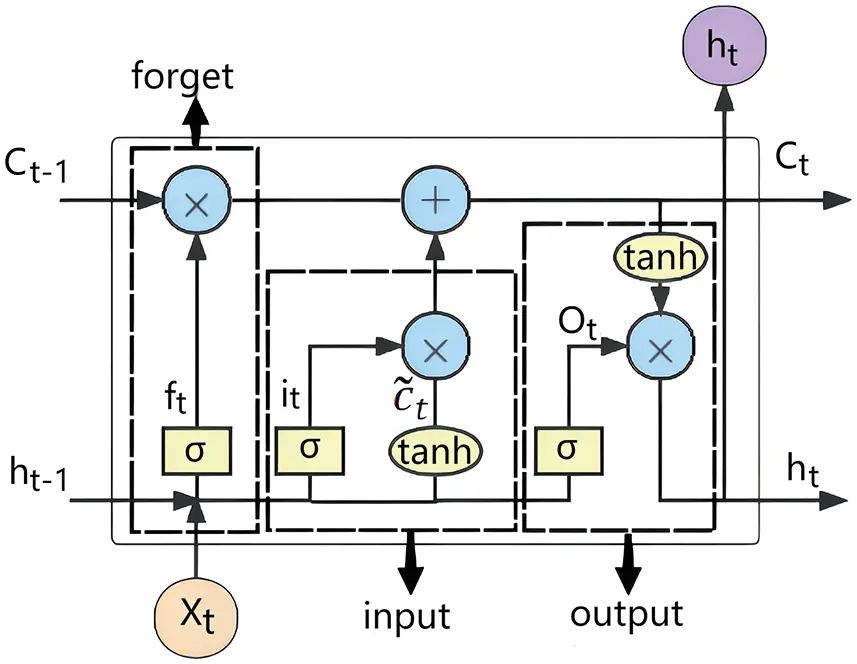Figure 1: LSTM network structure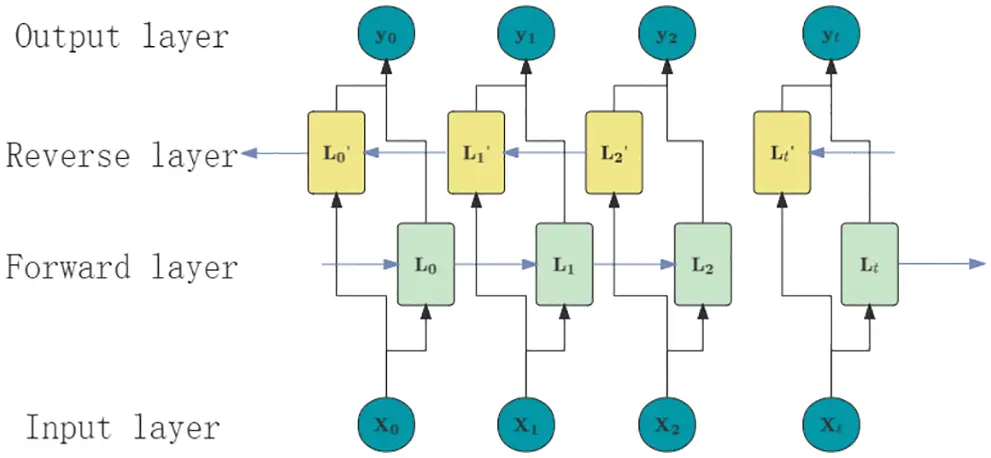Figure 2: BiLSTM network structure

2.3 Temporal Pattern Attention Mechanism

An attention mechanism is a resource allocation mechanism that mimics the attention of the human brain. In general, the human brain focuses its attention on the areas of interest at a particular moment, reducing or even eliminating the attention paid to other areas to obtain more detailed information that needs to be focused on, thereby suppressing other useless information, ignoring irrelevant information and amplifying the required information . The pre-attention and the post-attention mechanisms are the two types of attention mechanisms. The former adaptively weight variables to focus on important information while reducing noise influence. The post-attention mechanism selects the critical information, assigns weight to the hidden state of the neural network output, and then constructs the full connection layer to obtain the prediction result. Since the traditional attention mechanism tends to choose relevant time steps for weighting, thus, it is impossible to determine the most influential variables for weighting if there are multiple variables in a fixed time step, which may affect the prediction accuracy.

TPA extracts important features from the row vectors of the BiLSTM hidden state matrix through multiple one-dimensional convolutional neural network (CNN) filters. Therefore, the model learns the interdependence between multiple variables within the same time step and across all previous times and sequences. Its structure is shown in Fig. 3.

The hidden state matrix ={htw,htw+1,,ht1} of BiLSTM and its matrix time is w. The row vector of the hidden state matrix represents the state of a single variable at all time steps, that is, the vector composed of hourly power load data, average temperature, maximum temperature, minimum temperature, relative humidity, and week type; while the column vector represents the state of a single time step, that is, all the eigenvectors contained in a certain day. As shown, by convolving H with k 1 × w convolution kernels, Hc is obtained, and m represents 29 characteristic values for daily input variables, including power load data, average temperature, maximum temperature, minimum temperature, relative humidity, and week type.

i,jc=l=1wi,(tw1+l)×Cj,Tw+l(5)

where Cj denotes the j{th} filter of length T; T represents the length of the data set processed by the attention mechanism and the value of w in this paper; Hi,j represents the result value of the action of the i{th} row vector and the j{th} convolution kernel, as shown in Eq. (5).

f(ic,ht)=(ic)waht(6)

ic is the row vector of Hc; Wa is the weight matrix of m × k. The sigmoid function is used for normalization to obtain the attention weight, which is convenient for selecting multiple variables. Attention weight ai defined as:

αi=Sigmoid(f(ic,ht))(7)

Each row in Hc is weighted sum by attention weight ai to get output Vt.

vt=i=1nαiic(8)

where n represents the feature dimension, that is the number of features of the input variables. Finally, Vt is fused with the output ht at the last moment, and the final predicted output yt1+Δ is obtained through a linear transformation:

ht,=whht+wvvt(9)

yt1+Δ=wh,×ht,(10)

where wh and wv represent the weight matrix corresponding to different variables, and Δ represents the prediction time scale of different prediction tasks.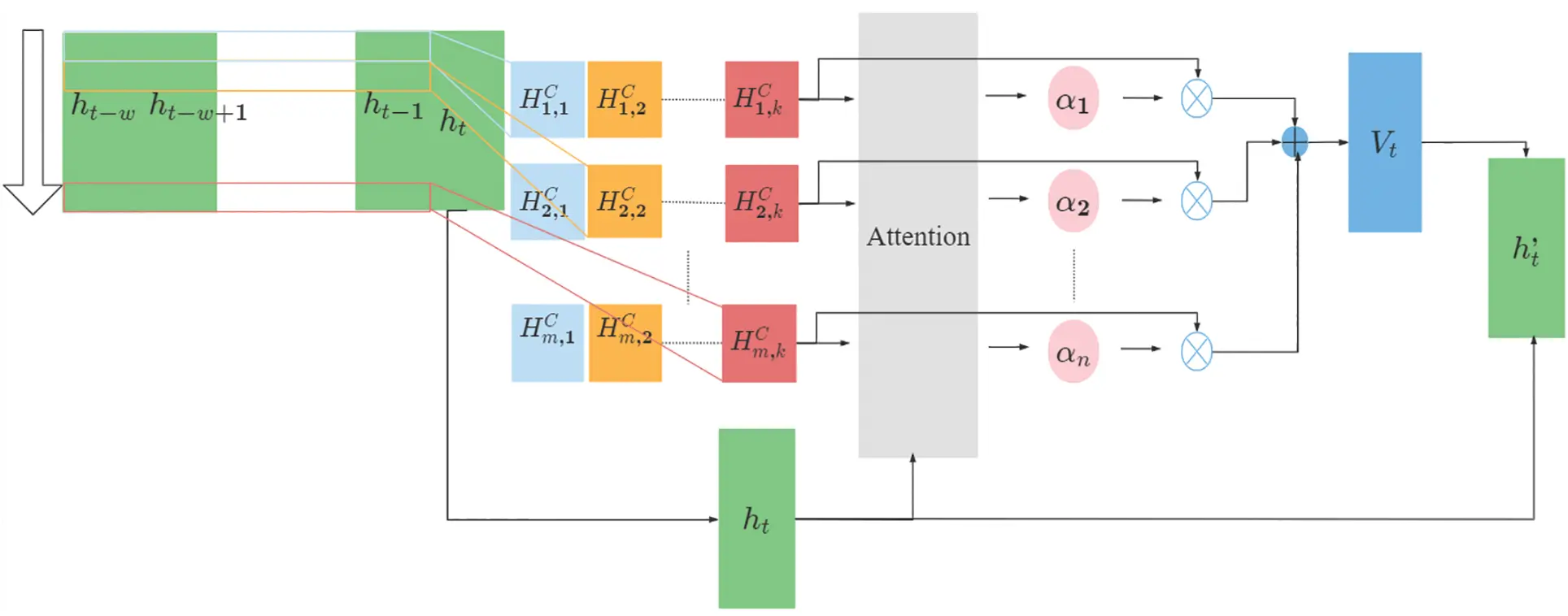Figure 3: TPA structure

2.4 The CSSA Sparrow Search Algorithm

The SSA sparrow search algorithm is a new swarm intelligence optimization algorithm proposed by Xue et al. [21,22]. Inspired by the sparrow foraging process, it aims to seek the optimal solution. Nevertheless, SSA may easily fall into the local optimum, which reduces the optimization efficiency. A CSSA algorithm introduces Tent chaotic search and Gaussian mutation based on SSA to solve SSA’s problem.

Tent chaotic sequence has small period and unstable period points, for which the variable rand(0,1)×1NT is introduced to improve the quality of the initial solution and enhance the global search ability of the algorithm without damaging the characteristics of chaotic variables. NT is the number of particles in the chaotic sequence, and rand (0, 1) is a random number between [0, 1]. In the process of population initialization of the SSA algorithm, the Tent chaotic sequence is introduced to initialize the population, and N D-dimensional vectors are generated. Each component is carried to the value range of the original problem space variable through Eq. (11).

Nnewd=dmin+(dmaxdmin)i(11)

where dmax and dmin are the maximum and minimum values of d{th} dimension variable Nnewd, respectively. Then chaotic disturbance is carried out on individuals according to Eq. (12).

Nnew,=(N,+Nnew)2(12)

where N, is the individual that needs chaotic disturbance, Nnew is the generated chaotic disturbance, and Nnew, is the individual after chaotic disturbance.

The Gaussian variation is derived from the Gaussian distribution. The original parameter values are replaced by a random number of the normal distribution with mean μ and variance σ2, as shown in Eq. (13).

mutation(x)=x×(1+N(0,1))(13)

where x is the original parameter value, N (0, 1) is the normal distribution random number with an expected value of 0 and a standard deviation of 1, and mutation(x) is the value after Gaussian variation. After one iteration of the SSA algorithm, the fitness value A of each sparrow and the average fitness value B of the sparrow population is recalculated. The sparrow is in the center position when A is less than B. The Gaussian mutation is carried out according to Eq. (13). If the mutated individual is better, it replaces the original one; otherwise, keep it unchanged. In other words, when A is larger than B, the sparrow is at the edge, and the chaotic perturbation is performed according to Eqs. (11) and (12). If the performance of the disturbed individual is better, replace it with the disturbed individual; otherwise, keep the original individual unchanged.

The optimization speed of the standard SSA algorithm is affected by the non-uniformity of logistic traversal, and the optimization efficiency will be reduced, while the value of the improved Tent map is more uniform. After adding Gaussian mutation, it can be seen from the normal distribution characteristics that the key search area of Gaussian mutation is a local area attached to the original individual, which is conducive to the algorithm to find the global minimum point efficiently and accurately and improves the robustness of the algorithm.

3  Short-Term Power Load Forecasting Model

In short-term load forecasting, the current load value is associated with the information of historical time and future time. In this paper, the BILSTM network considering bidirectional time information is selected as the underlying model of short-term load forecasting. TPA is introduced to compensate for the traditional attention mechanism that failed to extract the interdependence between multiple variables at different times and sequences. Also, to find the optimal hyperparameters, CSSA is introduced to optimize the hyperparameters of the model while constructing the model because hyperparameters are essential in the prediction model during the prediction process.

Herein, the minimized mean square error between the expected output and the actual output of the BiLSTM-TPA network is used as the fitness function, that is, to find a set of network hyperparameters to minimize the error of BiLSTM-TPA. The BiLSTM-TPA model structure is shown in Fig. 4, and the whole optimization process is shown in Fig. 5. BiLSTM-TPA optimized by CSSA algorithm is divided into input part, CSSA part, BiLSTM part, TPA part, and output part. The input part performs grey correlation analysis on the original dataset and selects the dataset similar to the day to be predicted. The BiLSTM part decodes the parameters introduced to obtain the number of iterations, learning rate, and the number of nodes in each hidden layer according to the principle of the CSSA algorithm. The TPA part selects relevant variables for weighting; the output part obtains the final prediction results, gets the error mean square deviation between the actual output value and the expected output value, and returns the mean square deviation as the fitness value to the CSSA part. The CSSA part performs the movement operations of predators, joiners, and alerters according to the fitness value and realizes the population update and the global optimum, by which the optimized network hyperparameters can finally be obtained.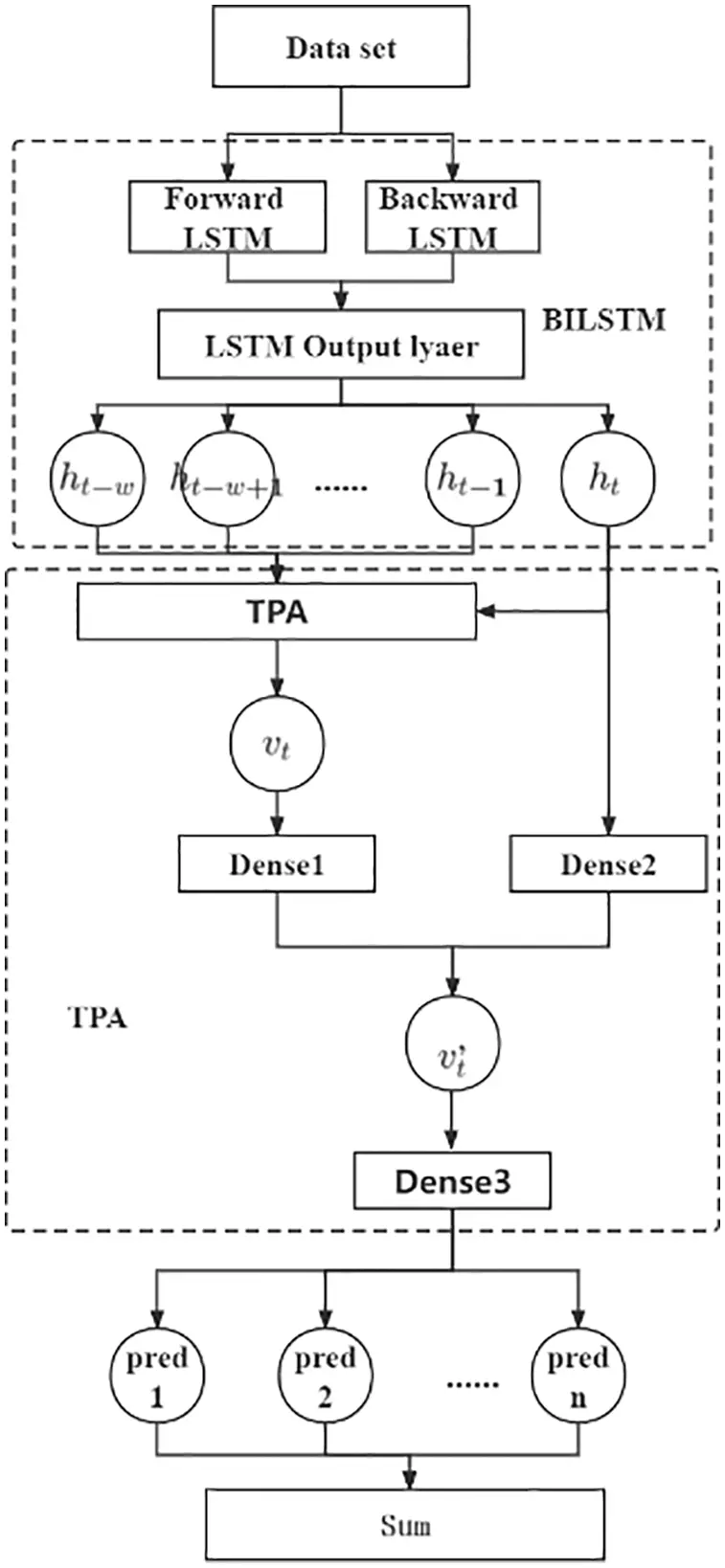Figure 4: BiLSTM-TPA model structure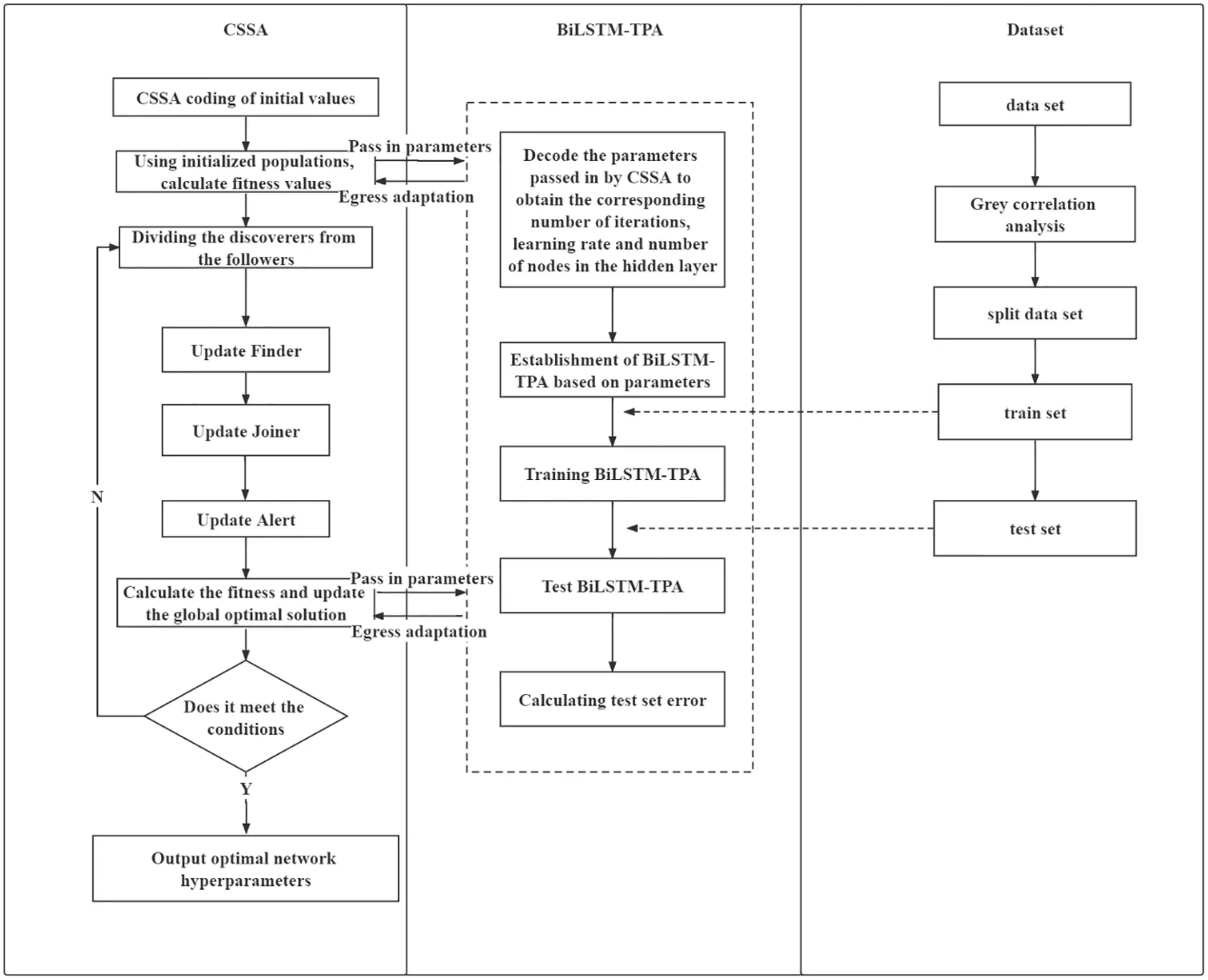Figure 5: Prediction model

3.1 The CSSA for the Optimization of BiLSTM-TPA Model

To optimize the super-parameters of BiLSTM, CSSA is introduced. Firstly, BiLSTM decodes the parameters introduced by CSSA to obtain the learning rate, the number of iterations, and the number of nodes in each hidden layer. After training the network model, the test set samples are predicted to obtain the error mean square deviation of the actual and expected output values. The mean square deviation is transmitted to the CSSA part as the fitness value, the optimal global solution is iteratively updated according to the fitness, and the optimized network model hyper-parameters are finally obtained. The chaotic sparrow search algorithm flow is shown in Fig. 6, and the specific steps are as follows: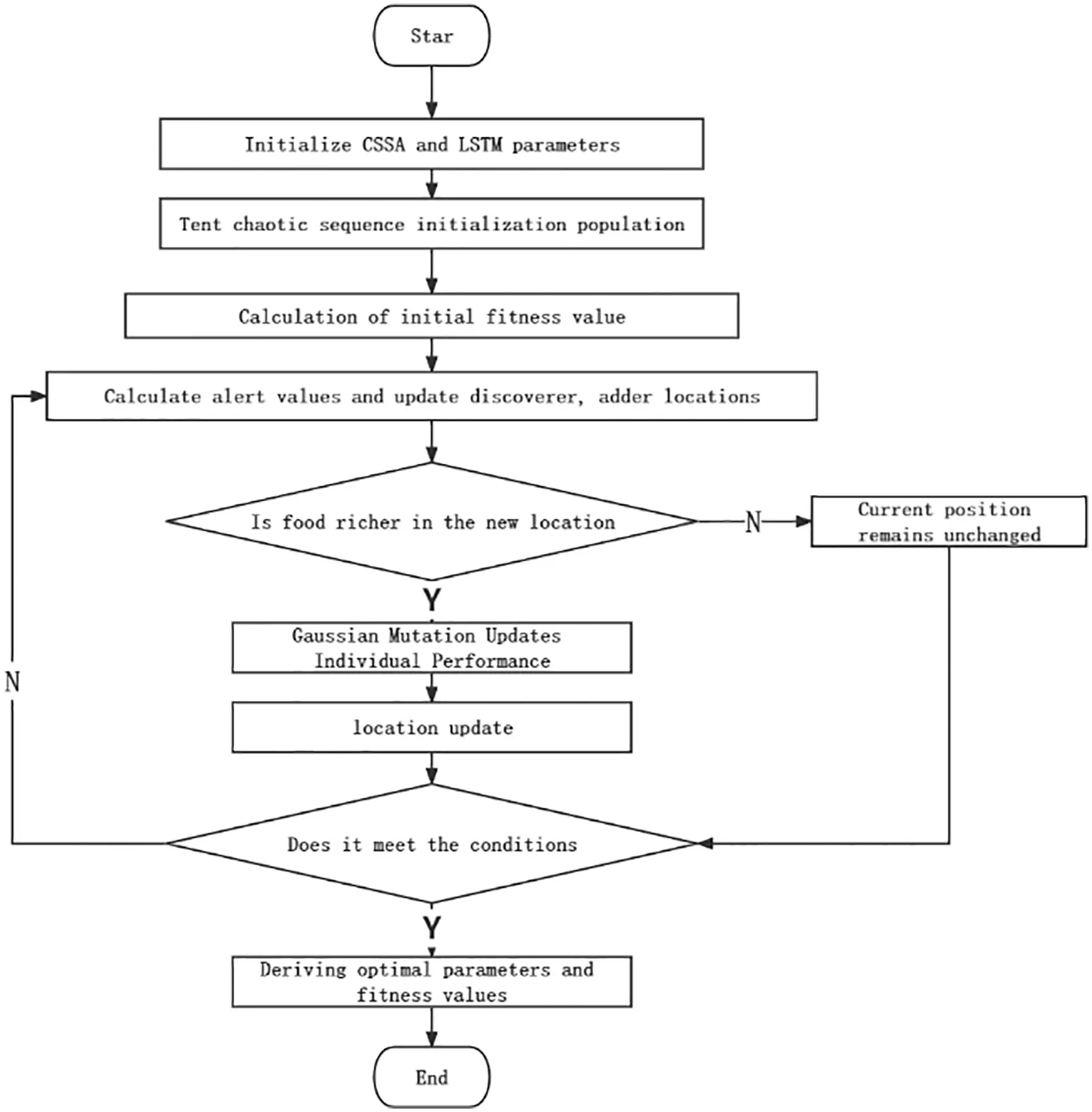Figure 6: CSSA optimization flowchart

Step 1: Initialize the population and the number of iterations, and initialize the proportion of predators and adders.

Step 2: Apply the Tent chaotic sequence in Section 2.4 to initialize the population.

Step 3: Calculate the fitness of each sparrow, and find the best position and fitness and the worst position and fitness.

Step 4: Select the top N sparrows with excellent fitness as discoverers, and the rest as the adder, and update the location of the discoverer and the adder according to SSA.

Step 5: Randomly select M sparrows for early warning and update the location.

Step 6: Update individual performance using Gaussian variation in Section 2.4.

Step 7: Update the position and fitness of the whole population to sort according to the current situation of the sparrow population.

Step 8: Determine whether the algorithm runs to meet the conditions and exit the output results if it is satisfied; otherwise, return to Step 4.

3.2 Evaluation Index

To assess the accuracy of the model, the mean absolute percentage error (MAPE), root mean square error (RMSE), mean absolute error (MAE), and determination coefficient (R2) are selected as the criteria for prediction accuracy in this paper, and their equations are calculated as follows:

MAPE=1ni=1n|yiyi^yi|×100%(14)

RMSE=1ni=1n(yiyi^)2(15)

MAE=1ni=1n|yiyi^|(16)

R2=1i=1n(yi^y¯)2i=1n(yiy¯)2(17)

where n represents the number of predicted samples, yi^ and yi correspond to the predicted and actual values of the corresponding points, respectively. MAPE can measure the prediction results, while RMSE and MAE can measure the prediction accuracy. The smaller the MAPE, RMSE, and MAE values, the higher the prediction accuracy. R2 is used to judge the quality of the model, and the value range is [0, 1]. The larger R2 is, the better the prediction results.

To demonstrate the validity of the model given in this paper, the results of LSTM, BiLSTM-Attention, BiLSTM-TPA, SSA-BiLSTM-Attention, CSSA-BiLSTM-Attention and IPSO-BiLSTM-Attention models are compared with the results of the method proposed in the paper.

4  Discussion

The validity of the proposed method is verified by using the measured data from February 13, 2010 to May 20, 2010, Zhejiang Province, and from May 01, 2021 to August 30, 2021, Shaanxi Province. The time interval of load data collected in Zhejiang Province is one h, and the original data set A is composed of meteorological factors collected by local weather stations. The time interval of load data collected in Shaanxi Province is 15 min, and the original data set B is composed of data preprocessing and meteorological factors collected by local meteorological stations.

4.1 Data Preprocessing and Input Feature Selection

The load data collected from Zhejiang Province is used as a sample to unify the unit of the load data collected in a certain area of Shaanxi Province. The data size of dataset A and the dataset B are composed of the load data of N × 24, the average temperature, the maximum temperature, the minimum temperature, the relative humidity, and the week type of the original data of N × 29, where N represents the total sampling time of the data. Meteorological factors can be obtained directly from the data collected by meteorological stations, and the week type is the degree of different coefficients obtained according to the electricity consumption at different weeks. To improve the training effect of the model, the linear mapping is used to calculate between [0, 1], as follows:

x=xxminxmaxxmin(18)

where x* is the normalized data, x is the original data, xmin is the minimum sample data, and xmax is the maximum sample data.

4.2 Data Prediction Analysis of Zhejiang Province

The dataset used is the standard dataset A of a certain place in Zhejiang Province. The average temperature, maximum temperature, minimum temperature, relative humidity, and week type of the day to be predicted on May 20, 2010 are used as reference values. The characteristics of the day to be predicted are taken as the characteristic sequence for grey correlation analysis. The data with a correlation degree greater than 0.7 are selected to form a dataset C similar to the day to be predicted. The correlation analysis is shown in Fig. 7.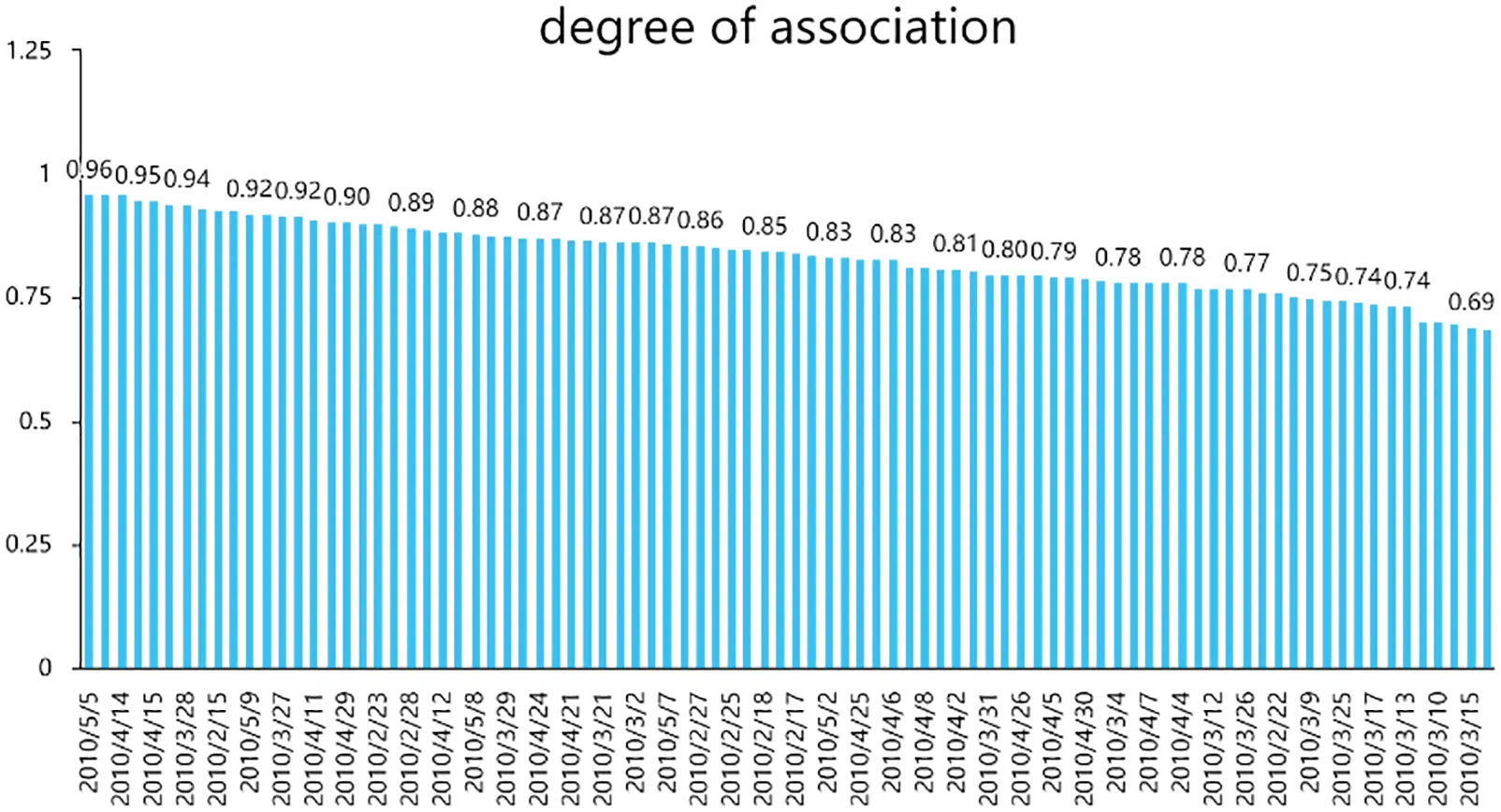Figure 7: Association of days to be predicted in Zhejiang Province

The training model is based on the data before May 18, 2010 in dataset C. The 29 data on May 19, 2010 and the average temperature, maximum temperature, minimum temperature, relative humidity, and week type on the predicted day were the input and output of the 24 loads on May 20, 2010. The CSSA parameter optimization is used to calculate the fitness calculation, and the mean square error of the validation set is used as a fitness function to find a set of parameters to minimize the network error.

The evaluation indexes of the prediction day are shown in Table 1. It can be seen that compared with the LSTM, BiLSTM-Attention, BiLSTM-TPA, SSA-BiLSTM-Attention CSSA-BiLSTM-Attention and IPSO-BiLSTM-AT models, MAPE of the proposed models is reduced by 1.59%, 1.01%, 0.94%, 0.27%, 0.18% and 0.85%, respectively, and RMSE is reduced by 1.218, 0.987, 0.718, 0.268, 0.178 and 0.543, respectively. The MAE index decreased by 1.178, 0.862, 0.596, 0.193, 0.179 and 0.611. The R2 index increased by 2.44%, 1.85%, 1.23%, 0.39%, 0.25% and 0.88%.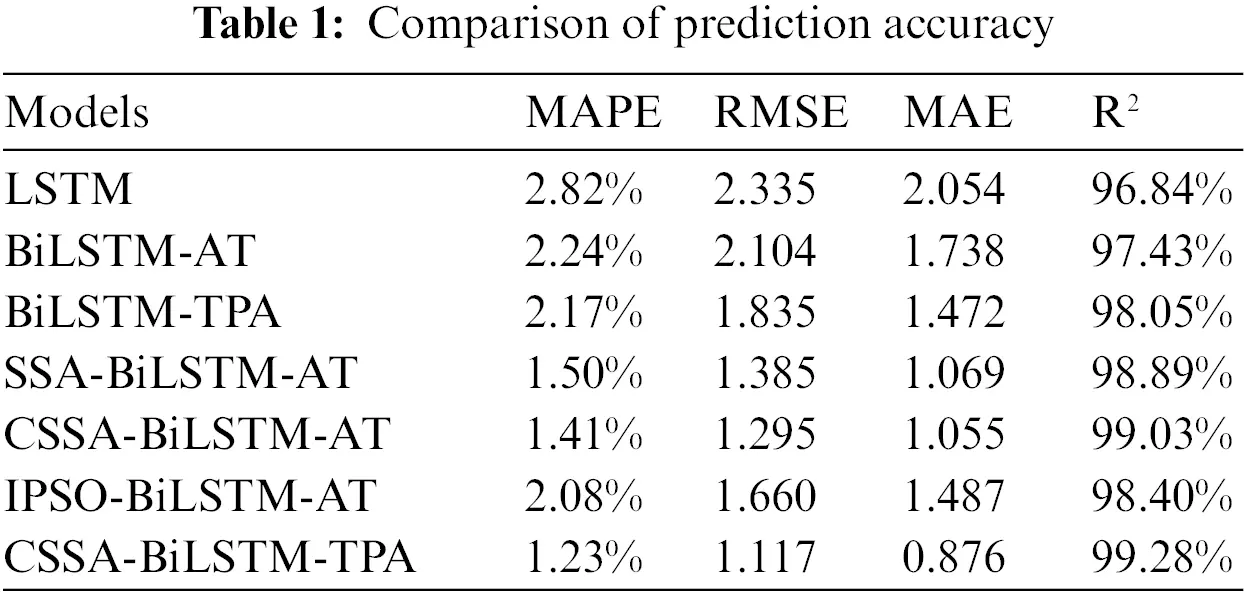To verify the improvement of the TPA mechanism in the short-term power load forecasting method, the BiLSTM-AT model based on the traditional attention mechanism and the BiLSTM-TPA model based on the time-mode attention mechanism were trained, respectively. The MAPE, RMSE, MAE, and R2 were used to evaluate the prediction accuracy under the same conditions. It was found that the MAPE, RMSE, and MAE of the BiLSTM-TPA model decreased by 0.07%, 0.269%, and 0.266%, respectively, and the R2 increased by 0.62%. As shown, the prediction accuracy of the TPA mechanism based on the BiLSTM model is higher than that of the traditional attention mechanism. Because different input variables have different characteristics, the traditional attention mechanism assigns the same attention weight to different characteristics of input variables and cannot consider the proportion of different variables in different time steps. On the other hand, the TPA mechanism performs feature extraction on the hidden row state matrix through the convolution layer, enabling the model to learn the interdependence between multiple variables within the same time step and across all previous times and sequences. Therefore, the TPA mechanism can select relevant information for each input variable from different time steps.

In the comparison of the CSSA-BiLSTM-AT model, SSA-BiLSTM-AT model, and BiLSTM-AT model, it is found that the MAPE, RMSE, and MAE of CSSA-BiLSTM-AT model and SSA-BiLSTM-AT model are significantly lower than those of BiLSTM-AT model, and the R2 is increased by 1.60% and 1.46%, respectively, indicating that the sparrow search algorithm and its improved algorithm have good results in the super-parameter optimization of BiLSTM-AT model. At the same time, comparing the CSSA-BiLSTM-AT model with the SSA-BiLSTM-AT model, the evaluation indicators MAPE, RMSE, and MAE are reduced by 0.09%, 0.090%, and 0.014%, respectively, and the R2 is increased by 0.14%, which verifies that the CSSA algorithm has higher optimization accuracy than the SSA algorithm. At last, Comparing the CSSA-BiLSTM-AT model with the IPSO-BiLSTM-AT model, the evaluation indicators MAPE, RMSE, and MAE are reduced by 0.85%, 0.543%, and 0.611%, respectively, and the R2 is increased by 0.88%. As verified, the proposed model has better performance than traditional prediction methods. The prediction results and real values of different models are shown in Fig. 8.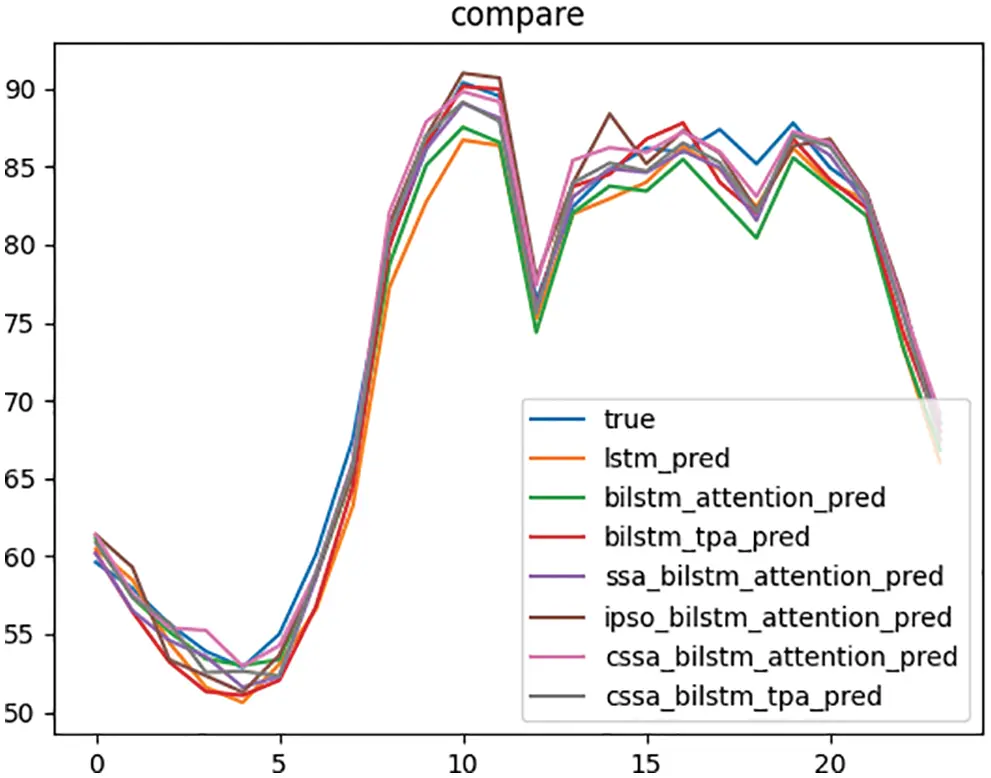Figure 8: Comparison of forecast results

4.3 Data Prediction Analysis of Shaanxi Province

To verify the applicability of our method, it is applied to the data of other provinces. The dataset used is the standard data set B of a certain place in Shaanxi Province. The average temperature, maximum temperature, minimum temperature, relative humidity, and week type on August 30, 2021 to be predicted are taken as the reference values, and the characteristics of its duration are taken as the characteristic sequence for grey correlation analysis. The data with a correlation degree greater than 0.7 are selected to form dataset D, similar to the day to be predicted.

CSSA optimizes the model hyper-parameters, and the fitness function is stabilized at 0.008 after iteration, as shown in Fig. 9a. The learning efficiency optimization curve is stable at 0.007 after iteration, as shown in Fig. 9b. The number of training optimization curves is stable at 238 after iteration, as shown in Fig. 9c. The number of nodes in the first and second hidden layers is stable at 50 and 23, respectively, as shown in Figs. 9d and 9e. The mean square error function is introduced herein as the fitness to CSSA, and the optimal global solution is iteratively updated according to the fitness value. Due to the introduction of chaotic disturbance and Gaussian mutation, CSSA has a stronger optimization ability than SSA. The standard SSA algorithm adopts a random point selection method in population initialization, so it is easily affected by the uneven ergodicity, while the improved Tent map has a more uniform value, so the algorithm can improve the quality of the initial solution and enhance the global search ability of the algorithm. After the iteration is completed, the fitness value of each sparrow and sparrow population is recalculated, and the individual is Gaussian mutated. A random number of the normal distribution is used to replace the original parameter value to search the regional focus near the original individual. Due to the characteristics of normal distribution, the search ability in the region near the original individual is enhanced, which is conducive to finding the global minimum point more accurately. After the final optimization by the above method, the network model after SSA optimization is better, with smaller fitness and stronger stability.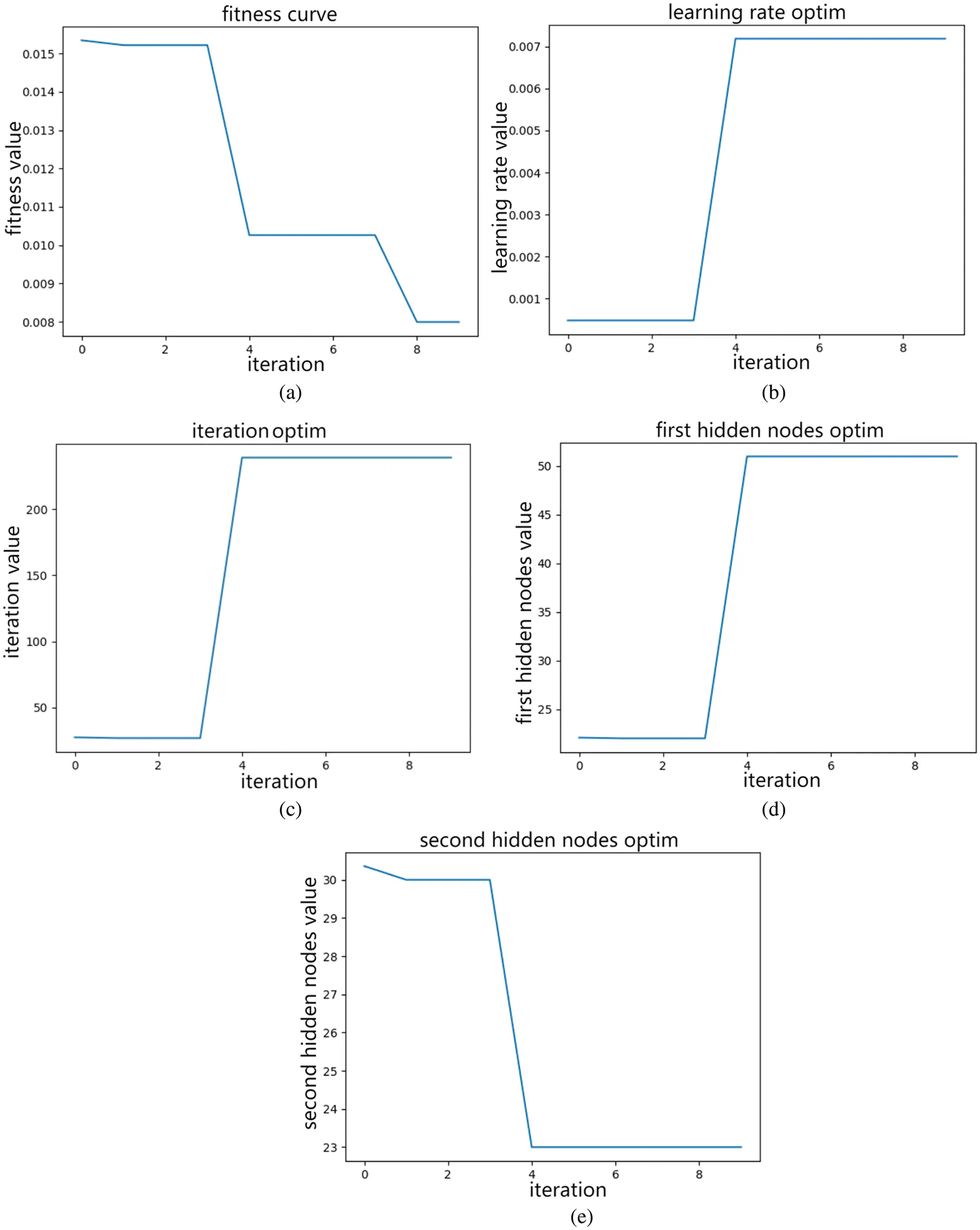Figure 9: (a) Adaptation convergence curve. (b) Learning rate optimisation curve. (c) Number of training sessions for the optimisation curve. (d) Node-seeking curve of the first implicit layer. (e) Node-seeking curve of the second implicit layer

The prediction results between the proposed method and other models are shown in Fig. 10. It can be seen from Fig. 10 that the prediction model results in this paper are closer to the real value. Compared with other methods, the proposed method has more accurate prediction results and can better show the variation law of load.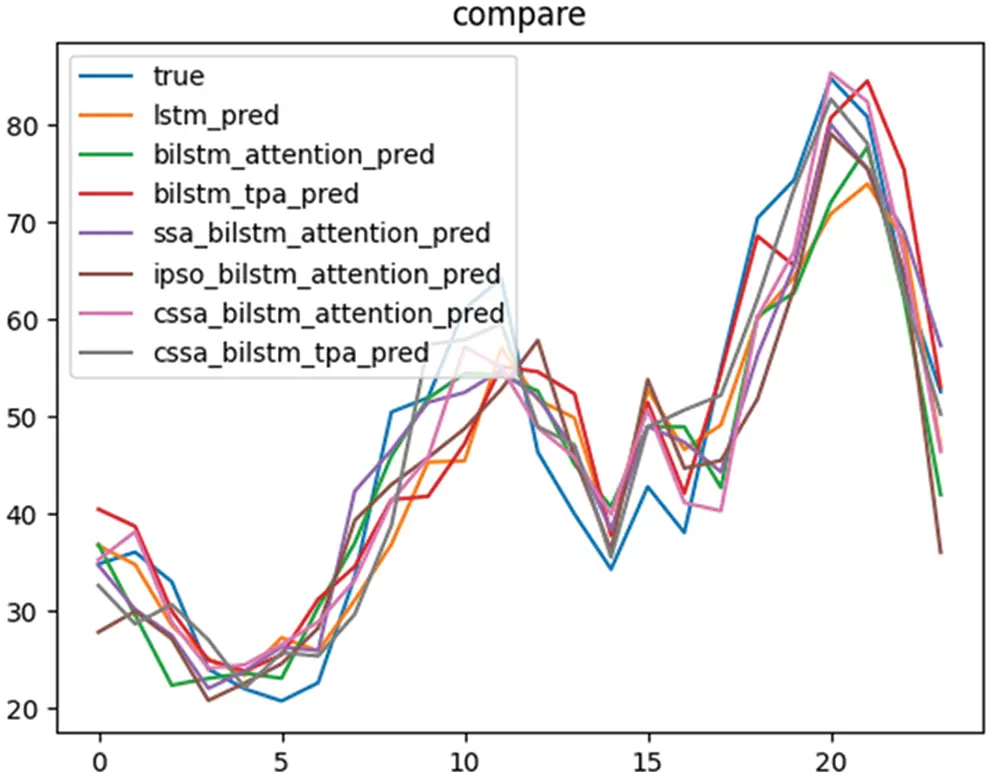Figure 10: Comparison of forecast results

To verify the rationality and stability of the proposed model, the evaluation indicators of each model are shown in Table 2. According to Table 2, the prediction accuracy of the model in this paper is the highest. Compared with the LSTM, BiLSTM-Attention, BiLSTM-TPA, SSA-BiLSTM-Attention, CSSA-BiLSTM-Attention and IPSO-BiLSTM-Attention models, the MAPE is reduced by 3.81%, 3.96%, 2.96%, 2.89%, 1.08% and 0.74%, respectively. While the RMSE index was reduced by 2.516, 2.191, 1.726, 1.409, 0.796, and 0.908, respectively. The comparison of MAE indicators decreased by 2.428, 2.153, 1.526, 1.625, 0.692, and 1.014. The R2 index was improved by 9.68%, 8.22%, 6.24%, 4.96%, 2.66% and 3.06%.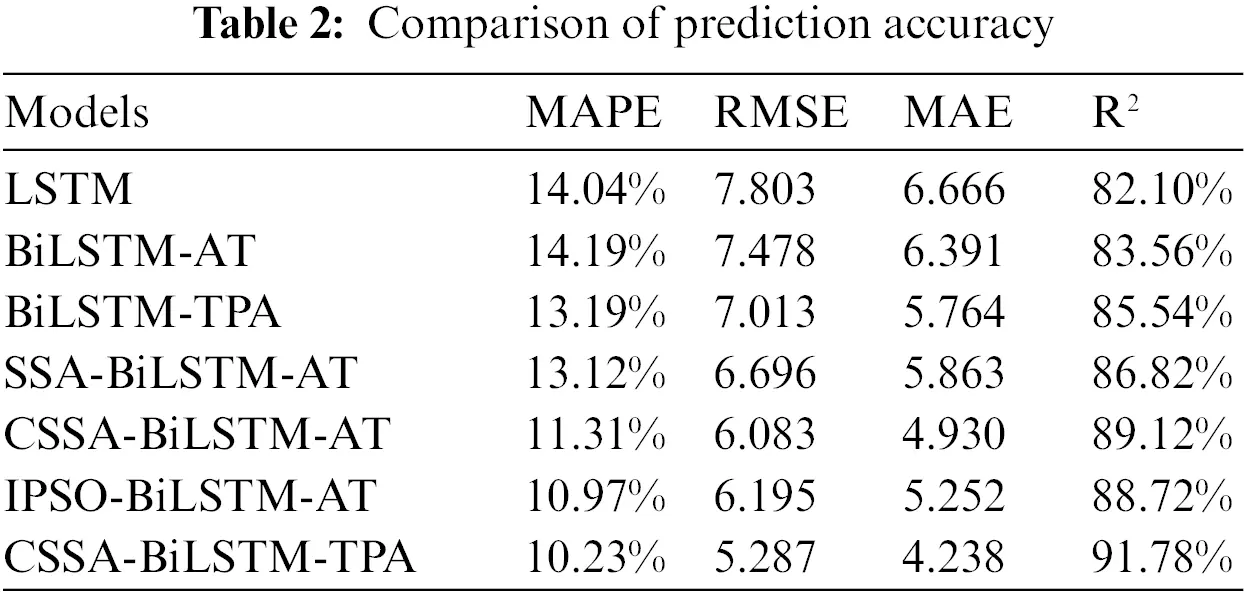Besides, we compared the BiLSTM-AT model based on the traditional attention mechanism and the BiLSTM-TPA model based on the temporal pattern attention mechanism to verify the versatility and stability of the TPA mechanism in short-term power load prediction methods under the same conditions. After training, MAPE, RMSE, MAE, and R2 were used as the evaluation indicators of prediction accuracy. It was found that compared with the BiLSTM-AT model, the MAPE, RMSE, and MAE of the BiLSTM-TPA model were reduced by 1%, 0.465%, and 0.627%, respectively, and the R2 was improved by 1.98%. The above data again verify that the prediction accuracy of the TPA mechanism based on the BiLSTM model is higher than that of the traditional attention mechanism.

The CSSA-BiLSTM-AT model, SSA-BiLSTM-AT model, and BiLSTM-AT model are found CSSA-BiLSTM-AT model and the SSA-BiLSTM-AT model have significantly lower MAPE, RMSE, and MAE, and the R2 is significantly improved compared with the BiLSTM-AT model. This shows the sparrow search algorithm’s applicability and stability and improved algorithm. By comparing the prediction evaluation indicators of the CSSA-BiLSTM-AT model and the SSA-BiLSTM-AT model, it is further verified that the CSSA algorithm has higher optimization accuracy than the SSA algorithm. At last, Compared with the IPSO algorithm, the CSSA algorithm has better prediction results in this paper.

5  Conclusions

This paper proposes a short-term power load forecasting method based on the chaotic sparrow search algorithm to optimize the time-series attention mechanism BiLSTM model. 1) Through the grey relational analysis method, the internal relationship of the data on the forecast day is analyzed, and the data sets similar to the forecast day are selected to reduce the difficulty of network processing and the interference caused by the noise data. Combined with the BiLSTM model, the bidirectional gated recurrent unit is used to fully extract the time series features, which further im-proves the prediction accuracy of the input information; 2) The TPA mechanism can use the convolution kernel to extract important features in the hidden feature matrix of BiLSTM. At the same time, considering the influence of different variables on the predictor variables, select relevant information from different time steps for different features, which is more efficient than traditional attention. Mechanism models bring higher prediction accuracy; 3) The improved particle swarm optimization algorithm IPSO is introduced to compare with the CSSA algorithm, which proves that the CSSA algorithm has better prediction accuracy in the prediction process of this paper. Compared with other prediction models, the CSSA-BiLSTM-TPA model is more accurate in-network hyperparameter optimization and can effectively improve the accuracy of short-term power load prediction under different data sets. Compared with other prediction models, this paper’s proposed method has better predictive performance on short-term power load forecasting tasks.

Funding Statement: This work has been supported by the Major Project of Basic and Applied Research in Guangdong Universities (2017WZDXM012). The authors would like to thank the anonymous reviewers, whose suggestions helped to substantially improve this manuscript.

Conflicts of Interest: The authors declare that they have no conflicts of interest to report regarding the present study.

## References

1. Wang, H., Zhang, Y., & Mao, H. (2019). Is based on the analysis of the characteristics of time and space variable data sharing car battery load forecasting method. Electric Power Automation Equipment, 33(12), 169-175. [Google Scholar] [CrossRef]
2. Liu, K., Ruan, J., Zhao, X., & Liu, G. (2021). Short-term load forecasting method for attention-GRU based on sparrow search optimization. Proceedings of the CSU-EPSA, 34(4), 99-106. [Google Scholar] [CrossRef]
3. Huang, Y., Zhu, J., Chen, M., & Chen, L. (2016). Power consumption prediction based on cubic exponential smoothing. Journal of Shanghai University of Engineering Science, 30(4), 365-369. [Google Scholar]
4. Chen, J., Hu, Z., Chen, W., Gao, M., & Du, Y. (2020). Load prediction of integrated energy system based on quadratic mode decomposition combination DBiLSTM-MLR. Automation of Electric Power Systems, 45(13), 85-94. [Google Scholar] [CrossRef]
5. Deng, D., Li, J., Zhang, Z., Teng, Y., & Huang, Q. (2019). Short-term power load forecasting based on EEMD-GRU-MLR. Power Grid Technology, 44(2), 593-602. [Google Scholar] [CrossRef]
6. Wang, Y., & Wu, M. (2018). Economy under the new normal medium and long-term load forecasting based on partial least-square regression model. Electric Power Automation Equipment, 38(3), 133-139. [Google Scholar] [CrossRef]
7. Kong, X., Zheng, F. E. Z., Cao, J., & Wang, X. (2018). Short-term load forecasting method based on deep belief network. Automation of Electric Power Systems, 42(5), 133-139. [Google Scholar]
8. Wang, Y., Dou, Y., & Meng, R. (2021). Short-term load forecasting model of MULTI-kernel neural network based on fuzzy C-means clustering, variational mode decomposition and swarm intelligence optimization. High Voltage Technology, 48(4), 1308-1319. [Google Scholar] [CrossRef]
9. Li, Z., Ye, L., Dai, B., Yu, Y., & Luo, Y. (2021). SSS based on IDSCNN-AM-LSTM combined neural network ultra-short-term wind power prediction method. High Voltage Technology, 48(6), 2117-2127. [Google Scholar] [CrossRef]
10. Li, C., Peng, X., Wang, H., Che, J., & Wang, B. (2021). SSS based on SDAE deep learning and multiple integration for short-term power prediction of wind power cluster. High Voltage Technology, 48(2), 504-512. [Google Scholar] [CrossRef]
11. Liu, Y., Peng, X., & Zheng, S. (2021). Short-term power load forecasting based on improved LS-SVM method research. Electric Measurement and Instrument, 58(5), 176-181. [Google Scholar] [CrossRef]
12. Liu, Y., Zhou, G., Liu, X., Wang, Y., & Zheng, Y. (2019). Based on intelligent recognition and similar day deviation correction method of short-term load forecasting. Power System Protection and Control, 47(12), 138-145. [Google Scholar] [CrossRef]
13. Wei, J., Zhao, H., Liu, D., Jia, H., & Wang, X. (2021). Short-term power load forecasting method based on attention mechanism of CNN-LSTM. Journal of North China Electric Power University (Natural Science Edition), 48(1), 42-47. [Google Scholar]
14. Shih, S., Sun, F., & Lee, H. (2019). Temporal pattern attention for multivariate time series forecasting. Machine Learning, 108(8–9), 1421-1441. [Google Scholar] [CrossRef]
15. Wang, Y., Shi, Y., Zhou, X., Zeng, Q., & Fang, B. (2022). BiLSTM ultra-short-term power prediction for muattentionti-wind turbines based on time-mode attention mechanism. High Voltage Technology, 48(5), 1884-1892. [Google Scholar] [CrossRef]
16. Wang, L. (2019). Application of improved bird swarm algorithm in image segmentation. China: Jiangxi University of Science and Technology Press.
17. Lv, X., Mu, X., Zhang, J., & Wang, Z. (2020). Chaos sparrow search optimization algorithm. Journal of Beijing University of Aeronautics and Astronautics, 47(8), 1712-1720. [Google Scholar] [CrossRef]
18. Li, W., Feng, F., & Jiang, Q. (2018). Prediction for railway passenger volume based on modified PSO optimized LSTM neural network. Journal of Rail Way Science and Engineering, 15(12), 3274-3280. [Google Scholar] [CrossRef]
19. Sun, G., Sun, X., Shan, Z., & Long, S. (2020). Prediction of groundwater PH value based on grey correlation and BP neural network. Mathematics in Practice and Cognition, 50(14), 147-155. [Google Scholar]
20. Li, Z., Ai, Q., Zhang, Y., & Xiao, F. (2019). A LSTM neural network method based on attention mechanism for ultra short-term load forecasting. Distribution & Utilization, 36(1), 17-22. [Google Scholar] [CrossRef]
21. Xue, J., & Shen, B. (2020). A novel swarm intelligence optimization approach: Sparrow search algorithm. Systems Science & Control Engineering, 8(1), 22-34. [Google Scholar] [CrossRef]
22. Huo, W., Zhou, J. (2021). Power load prediction model based on long short term memory and sparrow search algorithm. Proceedings of the 2nd International Conference on Advanced Electrical and Energy Systems (AEES), Tokyo, Japan. DOI 10.1088/1742-6596/2022/1/012018. [CrossRef]

Wen, J., Wang, Z. (2023). Short-Term Power Load Forecasting with Hybrid TPA-BiLSTM Prediction Model Based on CSSA. CMES-Computer Modeling in Engineering & Sciences, 136(1), 749–765.This work is licensed under a Creative Commons Attribution 4.0 International License , which permits unrestricted use, distribution, and reproduction in any medium, provided the original work is properly cited.

View

Like

## Related articles

• Romero-Castillo PA, MC Pérez Amador...
• Tianci Xia, Yuan Sun, Xiaobing...
• Delaram Molkara, Jose A. Silva...
• Pingping Xia, Aihua Xu, Tong Lian
• Yongjin Hu, Yuanbo Guo, Junxiu...